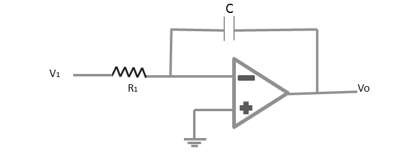# Semiconductor Devices - Integrator

The following figure shows that the feedback component used is a capacitor and the resulting connection is called as an integrator.The virtual-ground equivalent shows that an expression for the voltage between the input and the output can be derived in terms of the current (I), from the input to the output. Recall that virtual ground means we can consider the voltage at the junction of R and XC to be ground (since Vi ≈ 0 V) however no current goes into the ground at that point. The capacitive impedance can be expressed as

$$X_C = \frac{1}{jwC} = \frac{1}{sC}$$

Where s = jw as in the Laplace notation. Solving equation for $V_o/V_i$ yields the following equation

$$I = \frac{V_1}{R_1} = \frac{-V_0}{X_c} = \frac{-\frac{V_0}{I}}{sC} = \frac{V_0}{V_1}$$

$$\frac{V_0}{V_1} = \frac{-1}{sCR_1}$$

It can be written in the time domain as

$$V_o(t) = -\frac{1}{RC}\int V_1(t)dt$$